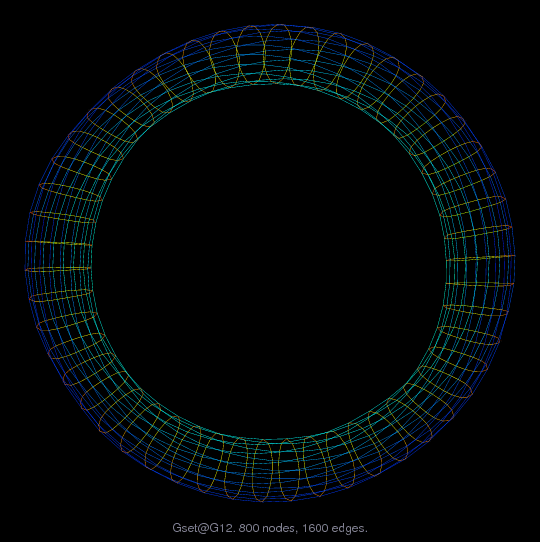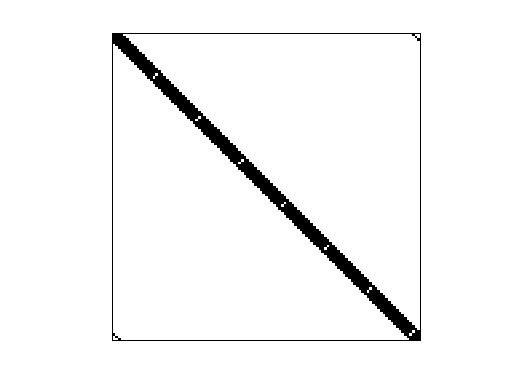Matrix: Gset/G12

Description: 2D torus, 16-by-50, uniformly random +1/-1 entries(undirected graph drawing)• Matrix group: Gset
• download as a MATLAB mat-file, file size: 6 KB. Use UFget(472) or UFget('Gset/G12') in MATLAB.

 Matrix properties number of rows 800 number of columns 800 nonzeros 3,200 # strongly connected comp. 1 explicit zero entries 0 nonzero pattern symmetry symmetric numeric value symmetry symmetric type integer structure symmetric Cholesky candidate? no positive definite? no

 author C. Helmberg editor Y. Ye date 1996 kind undirected weighted random graph 2D/3D problem? no

 SVD-based statistics: norm(A) 3.55611 min(svd(A)) 0.00521932 cond(A) 681.336 rank(A) 800 null space dimension 0 full numerical rank? yes

 singular values (MAT file): click here SVD method used: s = svd (full (A)) ; status: ok